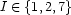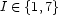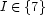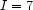## 6.1 Constraint Store

Logic Programming (henceforth LP) has the notion of a logic variable which is either free or bound to a value. Constraint Programming (henceforth CP) has the more general notion of a constrained variable, i.e. a variable that is no longer free, but whose value is not yet fully determined. For example, we may have the following partial information about an integer variable:meaning thatmay take only one of these 3 values. Such a piece of information is called a basic constraint. Thus, the LP idea of a set of bindings is replaced in CP by a set of basic constraints which we call the constraint store.

In LP, when a variable becomes bound to a value, this binding cannot subsequently be changed (except on backtracking): only new bindings may be added. Similarly in CP, the constraint store grows and the information therein improves monotonically. For example,might improve to become. When only one possible value remains, e.g., we say that the variable is determined and its value is 7, which we also write.

In (concurrent) CP, computations operate over a shared constraint store. The basic operations are ask and tell. A computation can ask whether a basic constraint is entailed (which happens when it or a stronger version of it is told to the store) or disentailed (i.e. its negation is entailed). The ask operation blocks until sufficient information has been concurrently accumulated in the store to answer the question either way. A computation can tell a basic constraint into the store (i.e. extend the store or improve the information already in the store, or derive a contradiction). The semantics of ask give us dataflow synchronization for free. Consider the statement:

case Lof nil then {P}[] H|T then {Q H T}end

it asks whether L=nil is entailed. As result, the statement blocks until there is sufficient information to answer the question one way or the other. In practice, this means that the statement blocks until L becomes bound.

Denys Duchier
Version 1.2.0 (20010221)